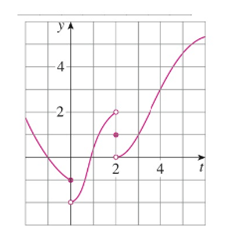Chapter 2.2, Problem 7E### Single Variable Calculus: Early Tr...

8th Edition
James Stewart
ISBN: 9781305270343

#### Solutions

Chapter
Section### Single Variable Calculus: Early Tr...

8th Edition
James Stewart
ISBN: 9781305270343
Textbook Problem

# For the function g whose graph is given, state the value of each quantity, if it exists. If it does not exist, explain why.(a) lim t → 0 − g ( t ) (b) lim t → 0 + g ( t ) (c) lim t → 0 g ( t ) (d) lim t → 2 − g ( t ) (e) lim t → 2 + g ( t ) (f) lim t → 2 g ( t ) (g) g ( 2 ) (h) lim t → 4 g ( t )(a)

To determine

To find: The value of limt0g(t).

Explanation

From the given graph, it is found that the curve move towards y=1 as t approaches 0 from the left, that is x<0

(b)

To determine

To find: The value of limt0+g(t).

(c)

To determine

To find: The value of limt0g(t).

(d)

To determine

To find: The value of limt2g(t).

(e)

To determine

To find: The value of limt2+g(t).

(f)

To determine

To find: The value of limt2g(t).

(g)

To determine

To find: The value of g(2).

(h)

To determine

To find: The value of limt4g(t).

### Still sussing out bartleby?

Check out a sample textbook solution.

See a sample solution

#### The Solution to Your Study Problems

Bartleby provides explanations to thousands of textbook problems written by our experts, many with advanced degrees!

Get Started

#### Solve the equations in Exercises 126. 1x9x3=0

Finite Mathematics and Applied Calculus (MindTap Course List)

#### Sketch the graph of the line that passes through the point (3, 2) and has slope 23.

Applied Calculus for the Managerial, Life, and Social Sciences: A Brief Approach

#### For Problems 11-18, evaluate each expression. (2.91)53.295

Mathematical Applications for the Management, Life, and Social Sciences